# How to combine columns without losing data

This post will guide you how to merge and combine multiple columns without losing any data in excel. How do I merge columns without losing data using Excel Formula. For example, you want to join two columns that contain first name and last name into one column without losing data.

If you want to merge multiple columns that you selected and you can use the “Merge & Center” command, but there is one problem is that it just keep the upper-left most data only. so if you just want to merge multiple columns and don’t care about if the data is deleted or not.

Firstly, Click “Merge & Center” command under Alignment group in Home Tab.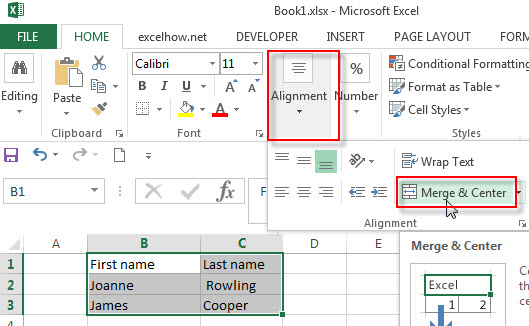then you will get a warning message that is “Merging cells only keeps the upper-left cell value, and discards the other values.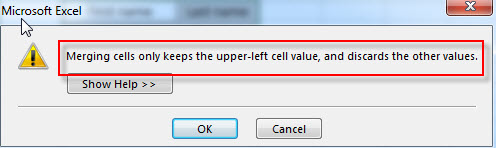## Merge columns without losing data

How to keep all data after merging columns. You can use the concatenate operator or CONCATENATE function to create an excel formula. Assuming that you want to merge column B and C into column D, you can use the following formulas:

`=B2&C2`

OR

`=CONCATENATE(B2, “ “ ,C2)`

You can write the above formula in Cell D2, you will see that both column B and column C have been merged into column D without losing any data.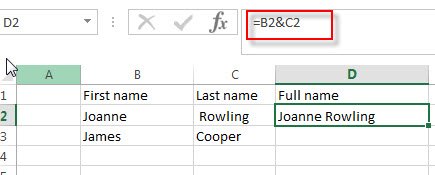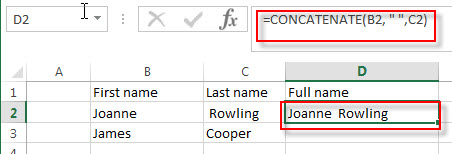Then you can drag the fill handle to other cells in Column D to apply the above formula to merge other cells.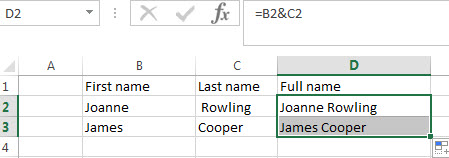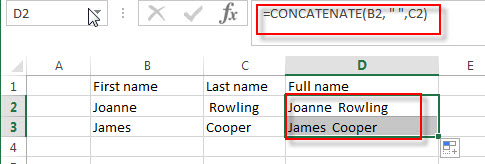### Related Functions

• Excel Concat function
The excel CONCAT function combines 2 or more strings or ranges together.This is a new function in Excel 2016 and it replaces the CONCATENATE function.The syntax of the CONCAT function is as below:=CONCAT (text1,[text2],…)…

### Related Posts

• Combine multiple workbooks into one workbook
If you want to combine multiple workbooks into one workbook, you need to open all workbooks, then determine the workbooks to merge and the destination of workbook.…
• Merge multiple worksheets into one worksheet
How to merge two or more excel worksheet into one worksheet by using some VBA code. You can create a new excel macro to combine multiple worksheets into one worksheet in Excel VBA…
Related Posts

Excel DVARP Function

This post will guide you how to use Excel DVARP function with syntax and examples in Microsoft excel. Description The Excel DVARP Function will get the variance of a population based on the entire population of numbers in a column ...

Excel DVAR Function

This post will guide you how to use Excel DVAR function with syntax and examples in Microsoft excel. Description The Excel DVAR Function will get the variance of a population based on a sample of numbers in a column in ...

Excel DSUM Function

This post will guide you how to use Excel DSUM function with syntax and examples in Microsoft excel. Description The Excel DSUM Function will add the numbers in a column or database that meets a given criteria. And so you ...

Excel DSTDEVP Function

This post will guide you how to use Excel DSTDEVP function with syntax and examples in Microsoft excel. Description The Excel DSTDEVP returns the standard deviation of a population based on the entire population of numbers in a column or ...

Excel DSTDEV Function

This post will guide you how to use Excel DSTDEV function with syntax and examples in Microsoft excel. Description The Excel DSTDEV returns the standard deviation of a population based on a sample of numbers in a column or database ...

Sidebar